gglikert(
data,
include = dplyr::everything(),
weights = NULL,
y = ".question",
variable_labels = NULL,
sort = c("none", "ascending", "descending"),
sort_method = c("prop", "mean", "median"),
sort_prop_include_center = totals_include_center,
exclude_fill_values = NULL,
labels_size = 3.5,
labels_color = "black",
labels_accuracy = 1,
labels_hide_below = 0.05,
totals_size = labels_size,
totals_color = "black",
totals_accuracy = labels_accuracy,
totals_fontface = "bold",
totals_include_center = FALSE,
totals_hjust = 0.1,
y_reverse = TRUE,
y_label_wrap = 50,
reverse_likert = FALSE,
width = 0.9,
facet_rows = NULL,
facet_cols = NULL,
facet_label_wrap = 50
)

gglikert_data(
data,
include = dplyr::everything(),
weights = NULL,
variable_labels = NULL,
sort = c("none", "ascending", "descending"),
sort_method = c("prop", "mean", "median"),
sort_prop_include_center = TRUE,
exclude_fill_values = NULL
)

gglikert_stacked(
data,
include = dplyr::everything(),
weights = NULL,
y = ".question",
variable_labels = NULL,
sort = c("none", "ascending", "descending"),
sort_method = c("prop", "mean", "median"),
sort_prop_include_center = FALSE,
labels_size = 3.5,
labels_color = "black",
labels_accuracy = 1,
labels_hide_below = 0.05,
y_reverse = TRUE,
y_label_wrap = 50,
reverse_fill = TRUE,
width = 0.9
)

## Arguments

data

a data frame

include

variables to include, accept tidy-select syntax

weights

optional variable name of a weighting variable, accept tidy-select syntax

y

name of the variable to be plotted on y axis (relevant when .question is mapped to "facets, see examples), accept tidy-select syntax

variable_labels

a named list or a named vector of custom variable labels

sort

should variables be sorted?

sort_method

method used to sort the variables: "prop" sort according to the proportion of answers higher than the centered level, "mean" considers answer as a score and sort according to the mean score, "median" used the median and the majority judgment rule for tie-breaking.

sort_prop_include_center

when sorting with "prop" and if the number of levels is uneven, should half of the central level be taken into account to compute the proportion?

exclude_fill_values

Vector of values that should not be displayed (but still taken into account for computing proportions), see position_likert()

should percentage labels be added to the plot?

labels_size

size of the percentage labels

labels_color

color of the percentage labels

labels_accuracy

accuracy of the percentages, see scales::label_percent()

labels_hide_below

if provided, values below will be masked, see label_percent_abs()

should the total proportions of negative and positive answers be added to plot? This option is not compatible with facets!

totals_size

size of the total proportions

totals_color

color of the total proportions

totals_accuracy

accuracy of the total proportions, see scales::label_percent()

totals_fontface

font face of the total proportions

totals_include_center

if the number of levels is uneven, should half of the center level be added to the total proportions?

totals_hjust

horizontal adjustment of totals labels on the x axis

y_reverse

should the y axis be reversed?

y_label_wrap

number of characters per line for y axis labels, see scales::label_wrap()

reverse_likert

if TRUE, will reverse the default stacking order, see position_likert()

width

bar width, see ggplot2::geom_bar()

facet_rows, facet_cols

A set of variables or expressions quoted by ggplot2::vars() and defining faceting groups on the rows or columns dimension (see examples)

facet_label_wrap

number of characters per line for facet labels, see ggplot2::label_wrap_gen()

add a vertical line at 50%?

reverse_fill

if TRUE, will reverse the default stacking order, see ggplot2::position_fill()

## Value

A ggplot2 plot or a tibble.

## Details

Combines several factor variables using the same list of ordered levels (e.g. Likert-type scales) into a unique data frame and generates a centered bar plot.

You could use gglikert_data() to just produce the dataset to be plotted.

If variable labels have been defined (see labelled::var_label()), they will be considered. You can also pass custom variables labels with the variable_labels argument.

vignette("gglikert"), position_likert(), stat_prop()

## Examples

library(ggplot2)
library(dplyr)
#>
#> Attaching package: ‘dplyr’
#> The following objects are masked from ‘package:stats’:
#>
#>     filter, lag
#> The following objects are masked from ‘package:base’:
#>
#>     intersect, setdiff, setequal, union

likert_levels <- c(
"Strongly disagree",
"Disagree",
"Neither agree nor disagree",
"Agree",
"Strongly agree"
)
set.seed(42)
df <-
tibble(
q1 = sample(likert_levels, 150, replace = TRUE),
q2 = sample(likert_levels, 150, replace = TRUE, prob = 5:1),
q3 = sample(likert_levels, 150, replace = TRUE, prob = 1:5),
q4 = sample(likert_levels, 150, replace = TRUE, prob = 1:5),
q5 = sample(c(likert_levels, NA), 150, replace = TRUE),
q6 = sample(likert_levels, 150, replace = TRUE, prob = c(1, 0, 1, 1, 0))
) %>%
mutate(across(everything(), ~ factor(.x, levels = likert_levels)))

gglikert(df)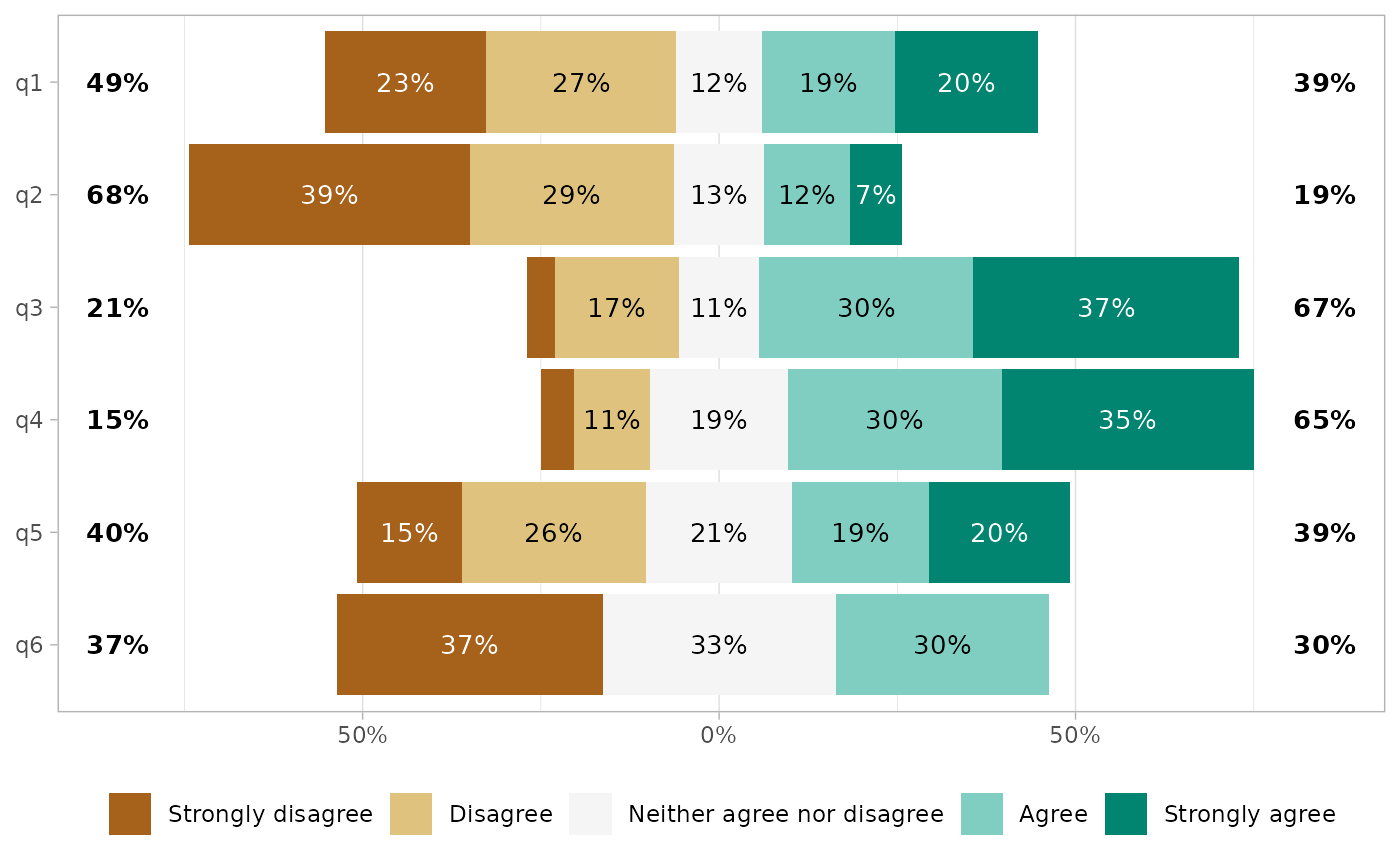gglikert(df, include = q1:3)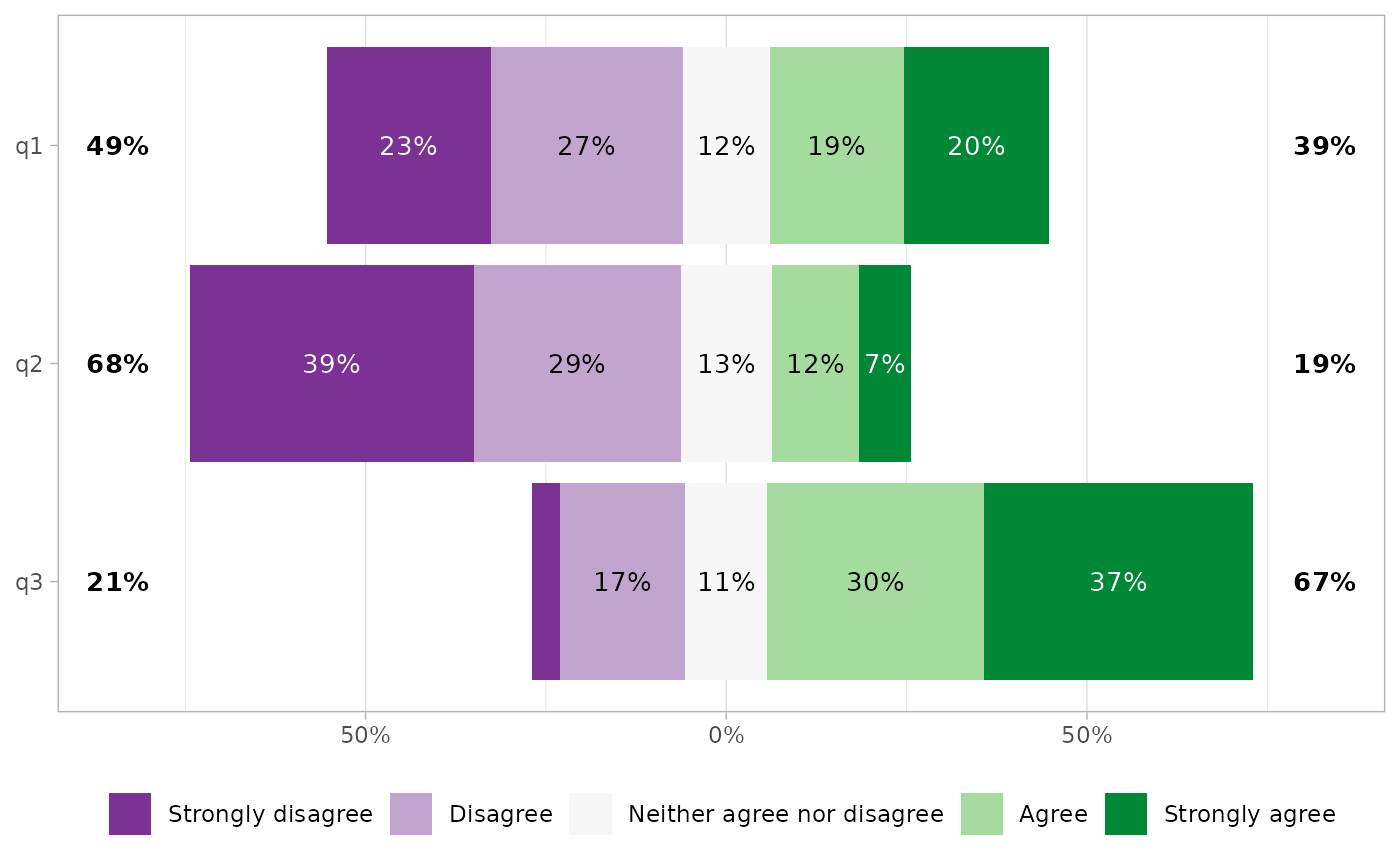gglikert(df, sort = "ascending")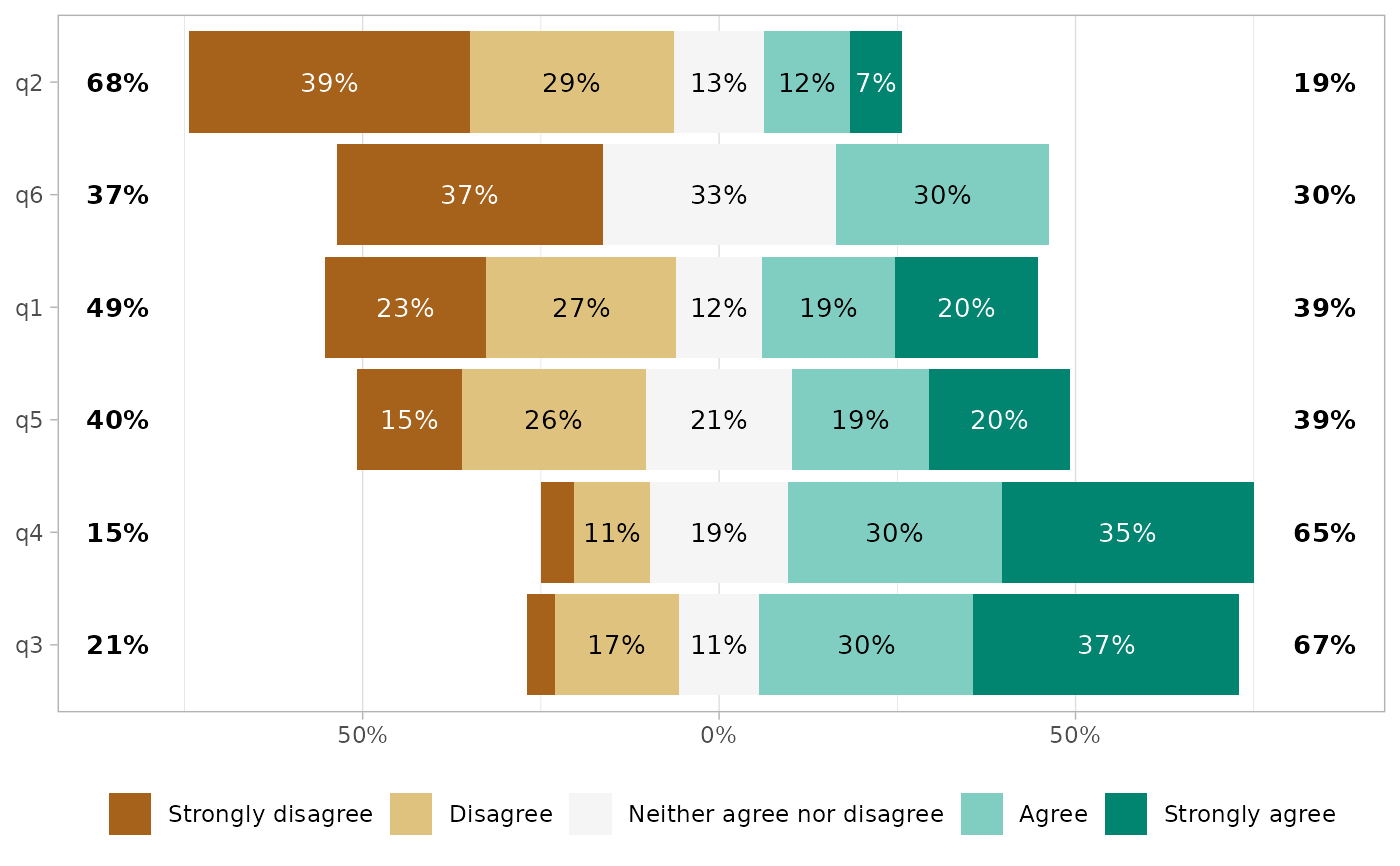# \donttest{
gglikert(df, sort = "ascending", sort_prop_include_center = TRUE)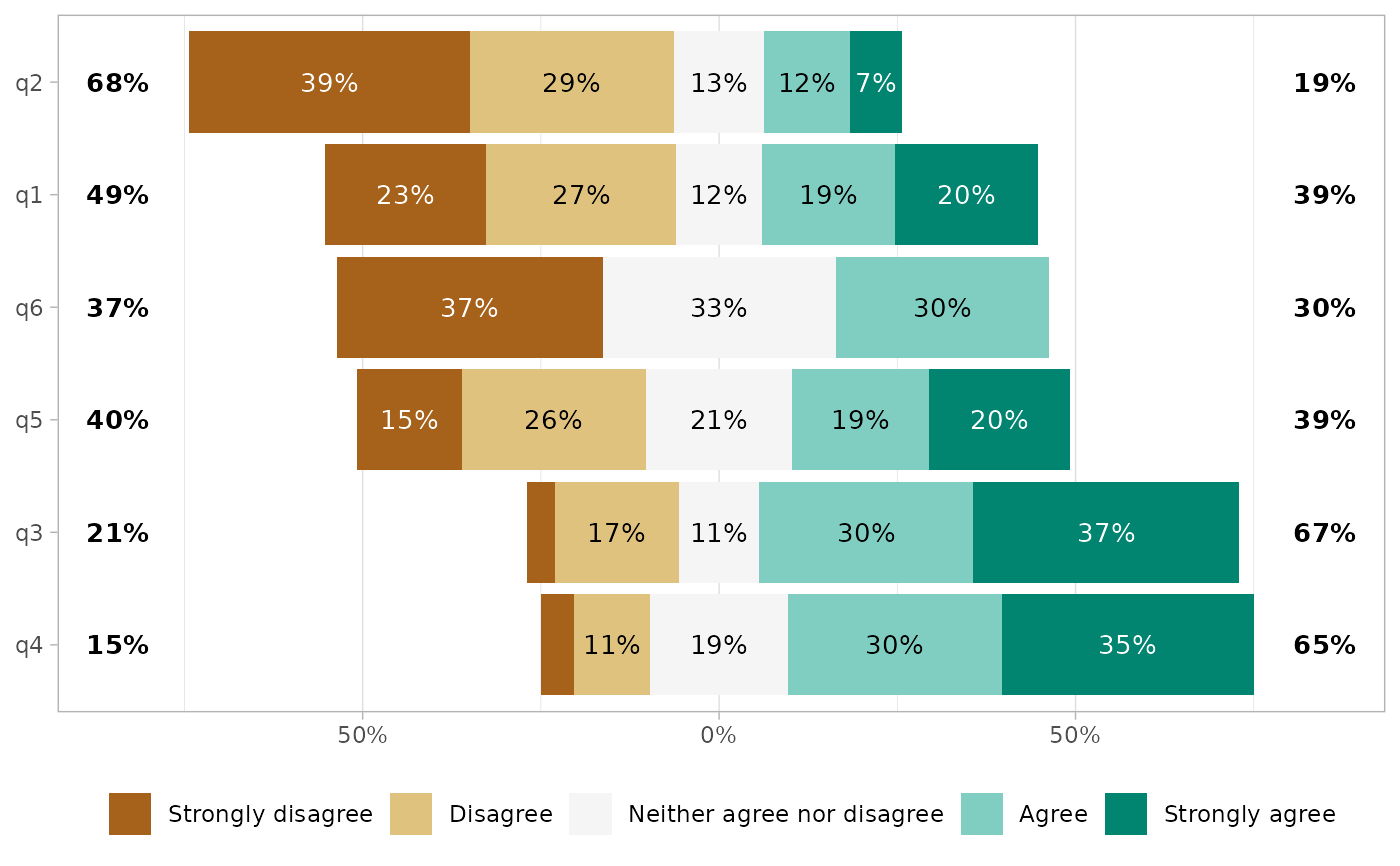gglikert(df, sort = "ascending", sort_method = "mean")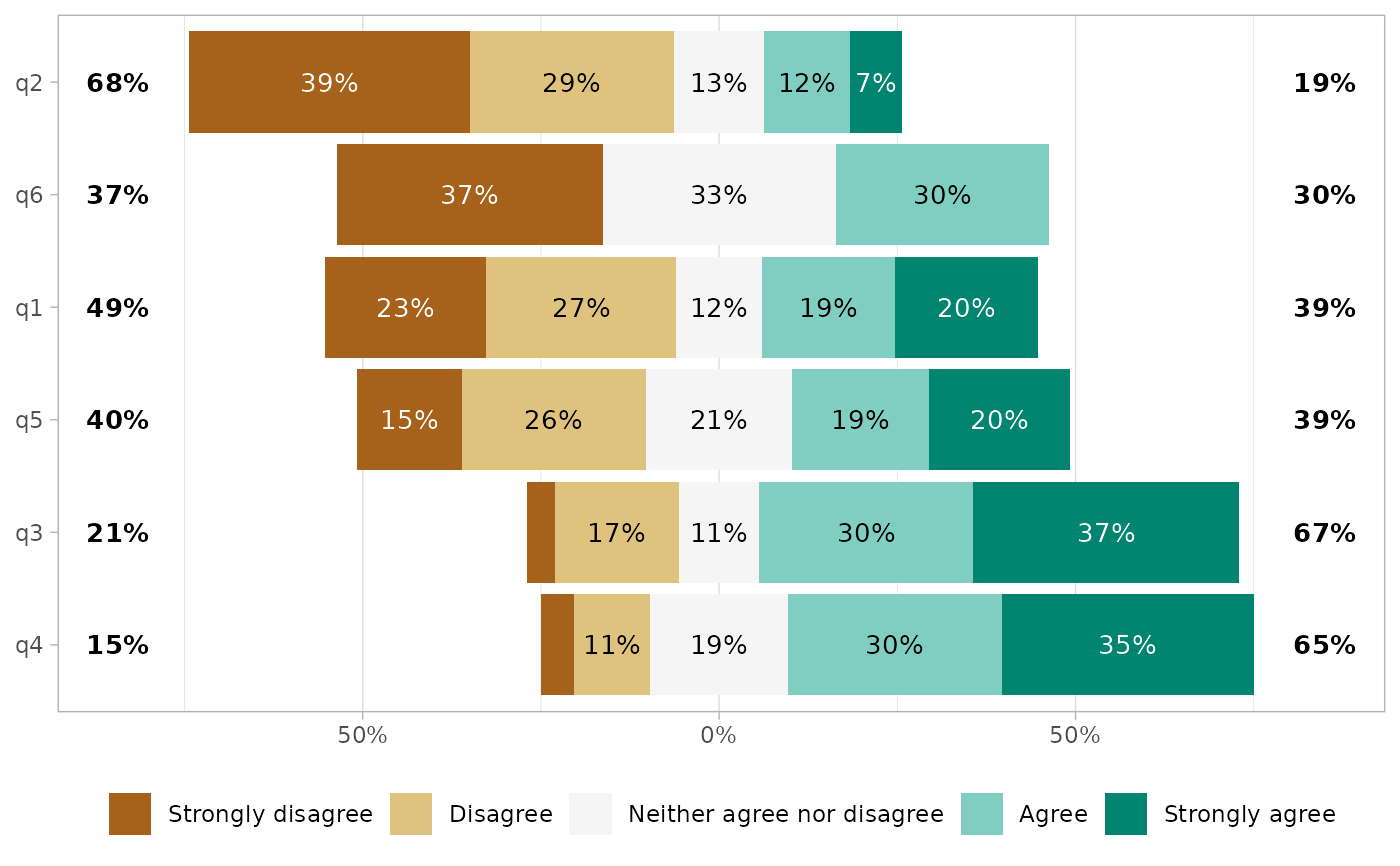gglikert(df, reverse_likert = TRUE)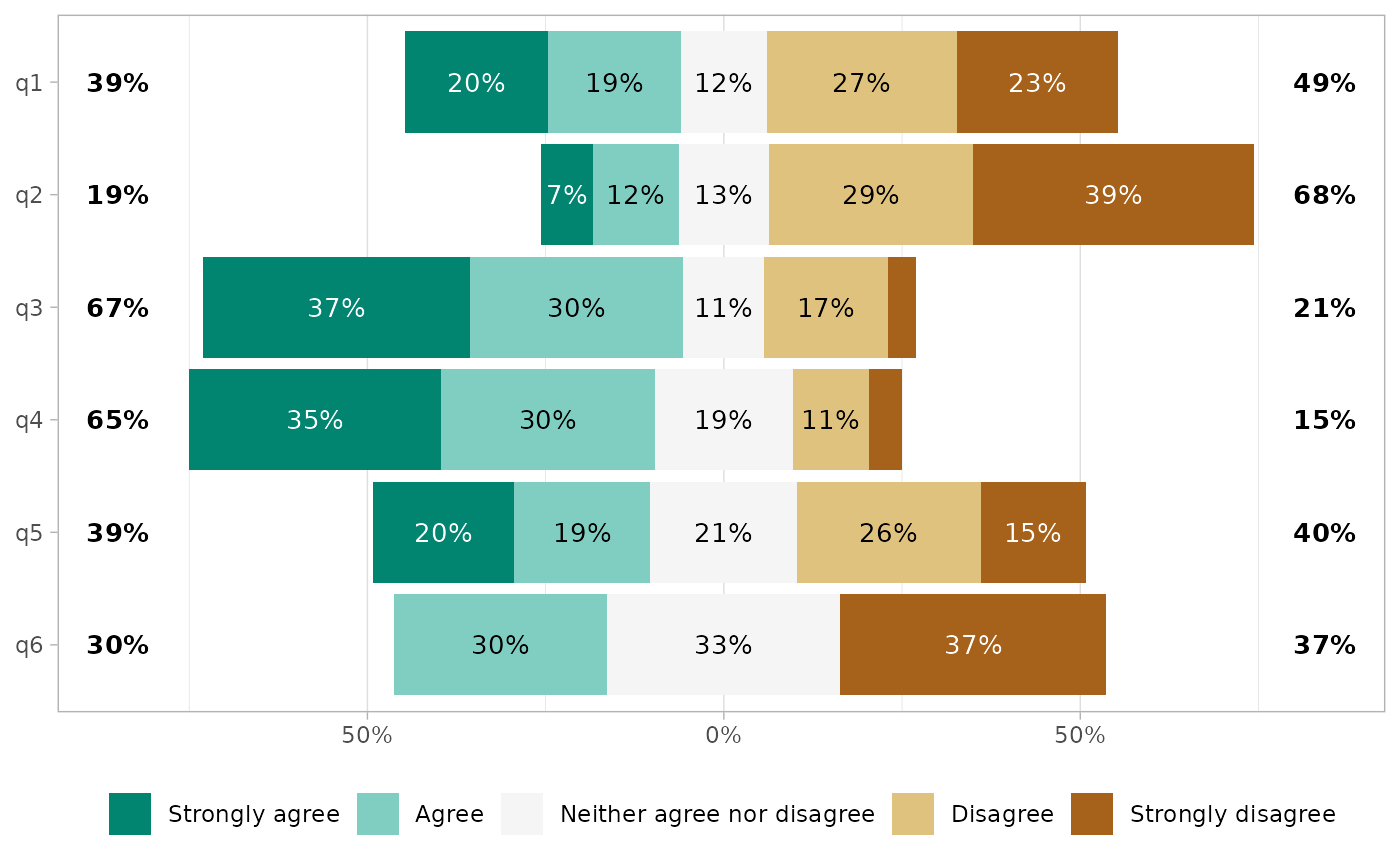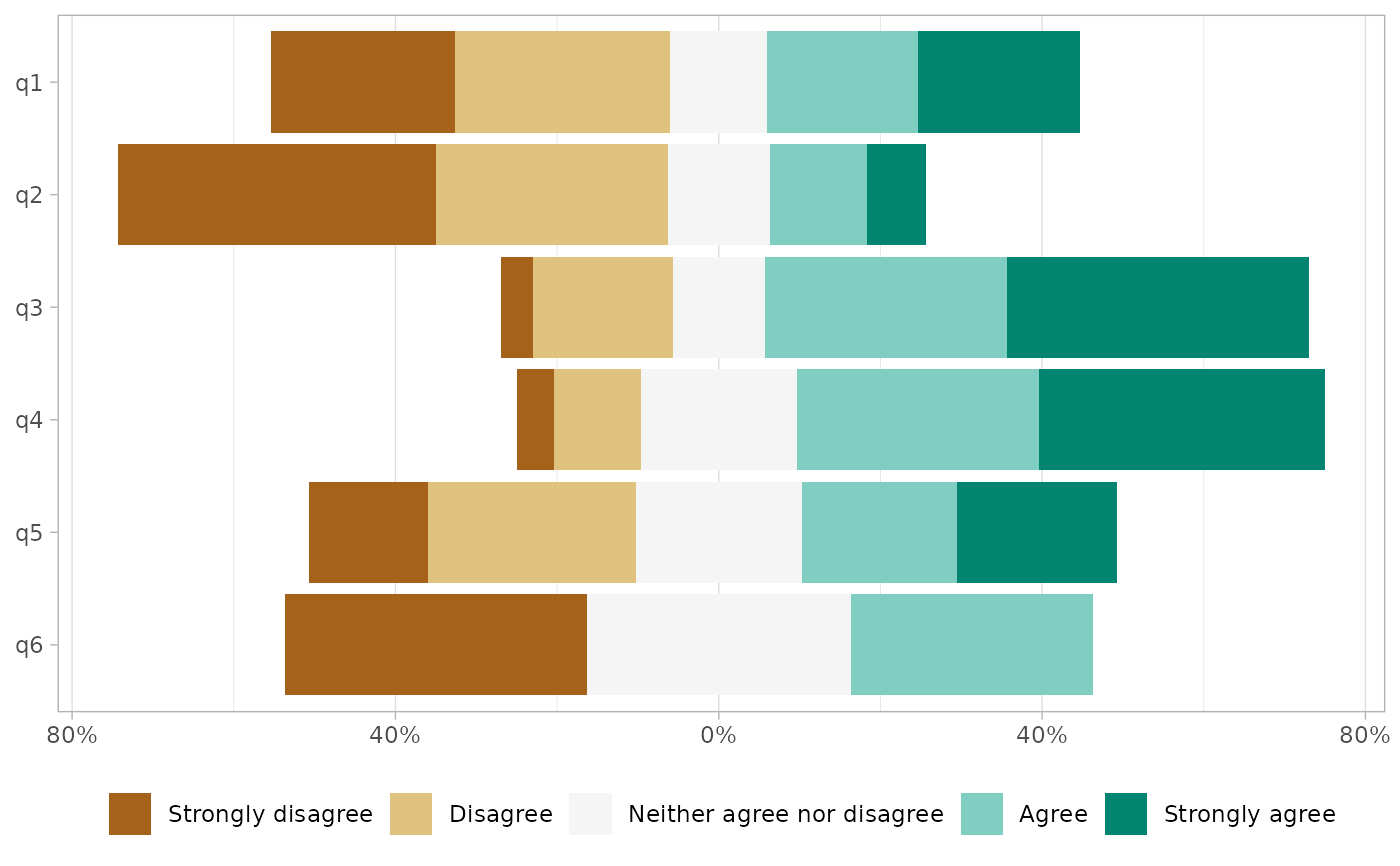gglikert(
df,
totals_include_center = TRUE,
totals_hjust = .25,
totals_size = 4.5,
totals_fontface = "italic",
totals_accuracy = .01,
labels_accuracy = 1,
labels_size = 2.5,
labels_hide_below = .25
)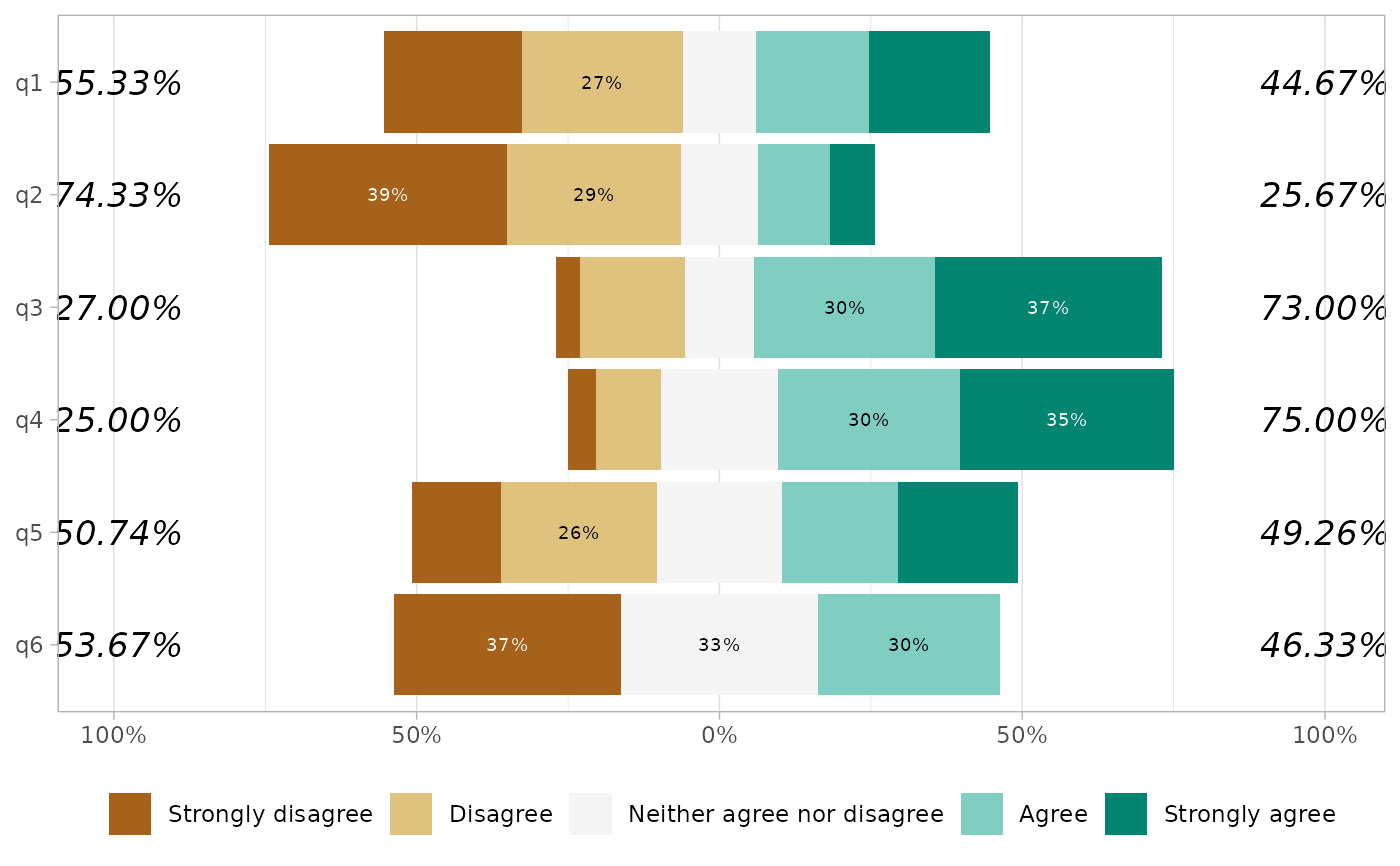gglikert(df, exclude_fill_values = "Neither agree nor disagree")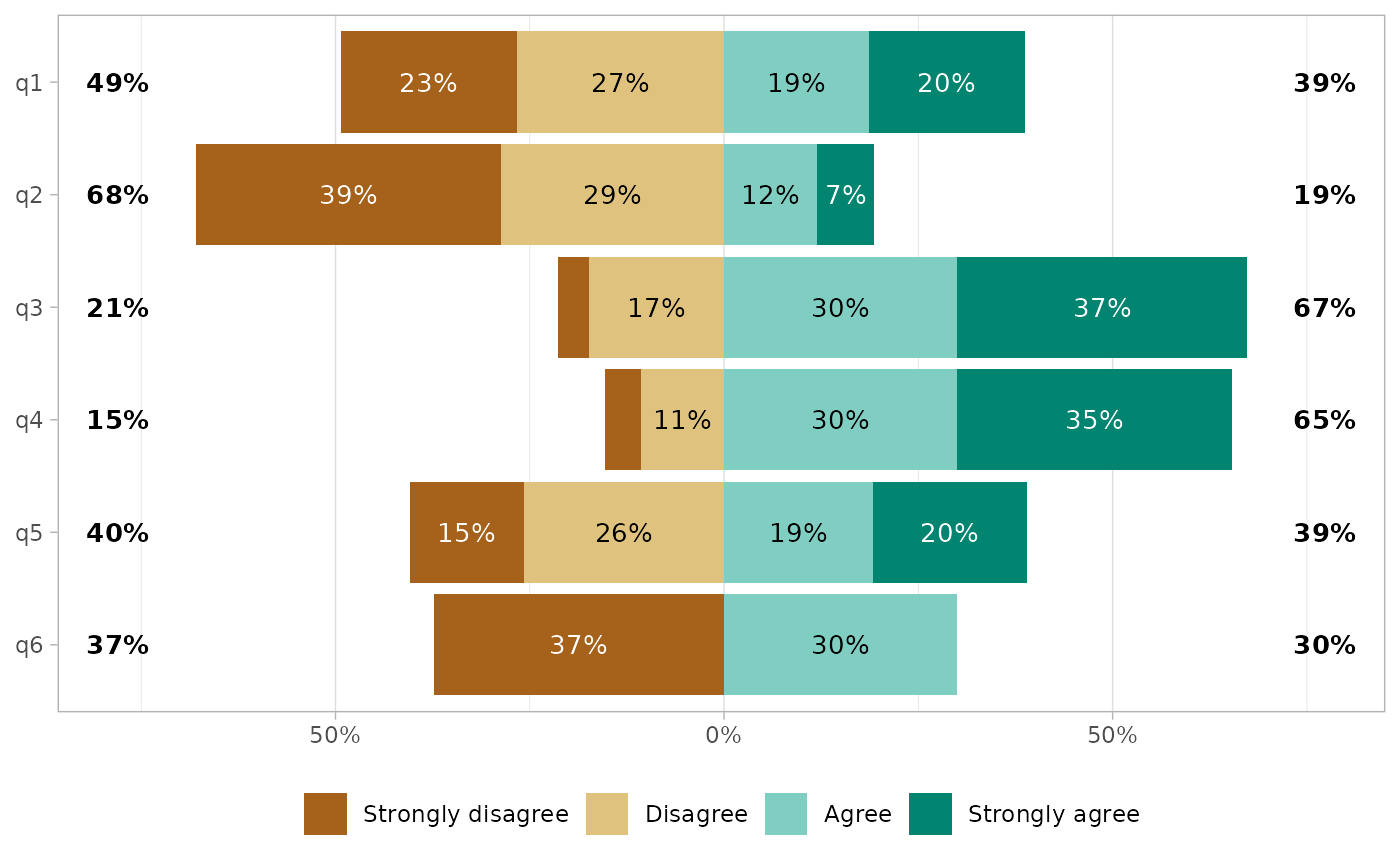if (require("labelled")) {
df %>%
set_variable_labels(
q1 = "First question",
q2 = "Second question"
) %>%
gglikert(
variable_labels = c(
q4 = "a custom label",
q6 = "a very very very very very very very very very very long label"
),
y_label_wrap = 25
)
}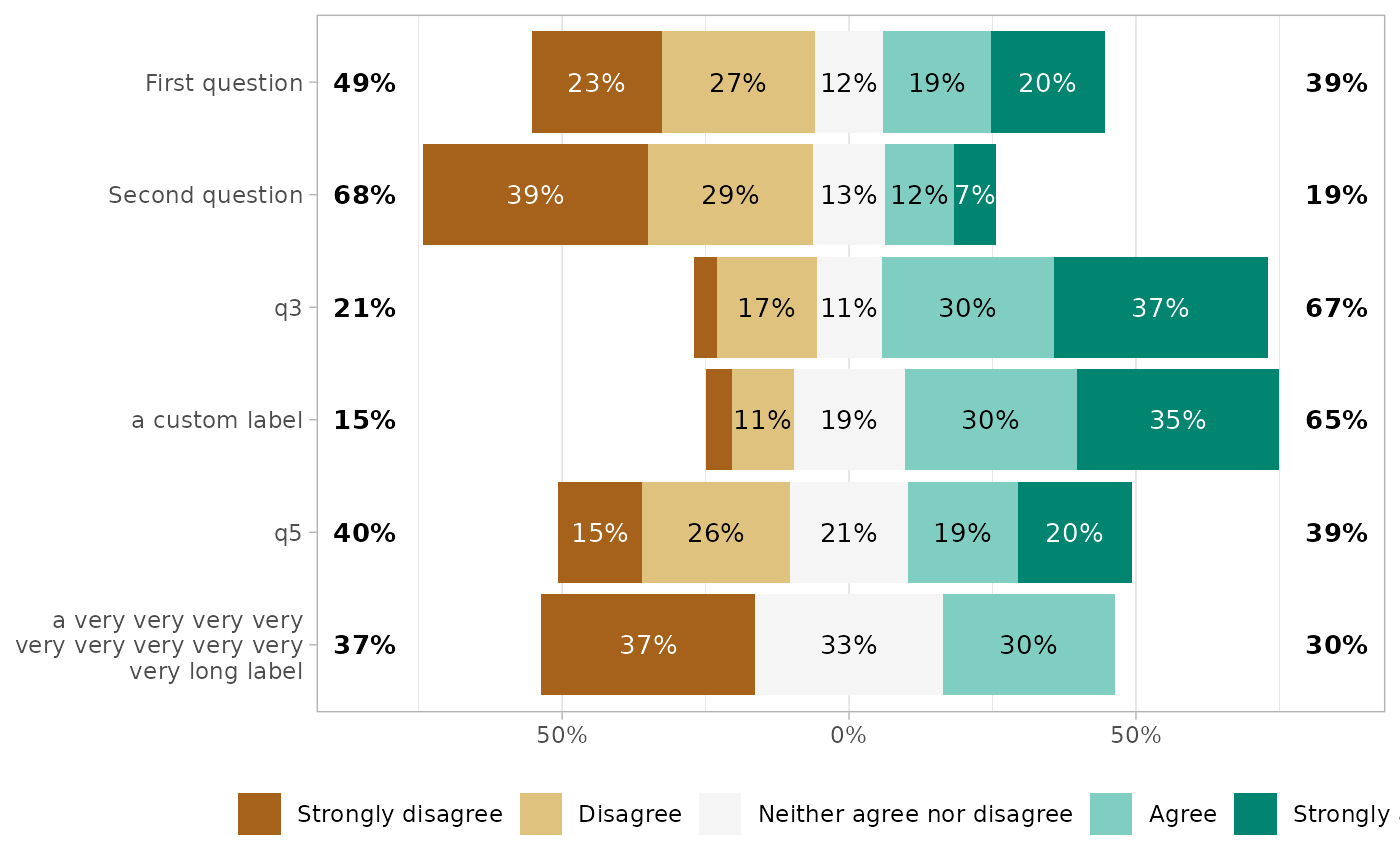# Facets
df_group <- df
df_group\$group <- sample(c("A", "B"), 150, replace = TRUE)

gglikert(df_group, q1:q6, facet_rows = vars(group))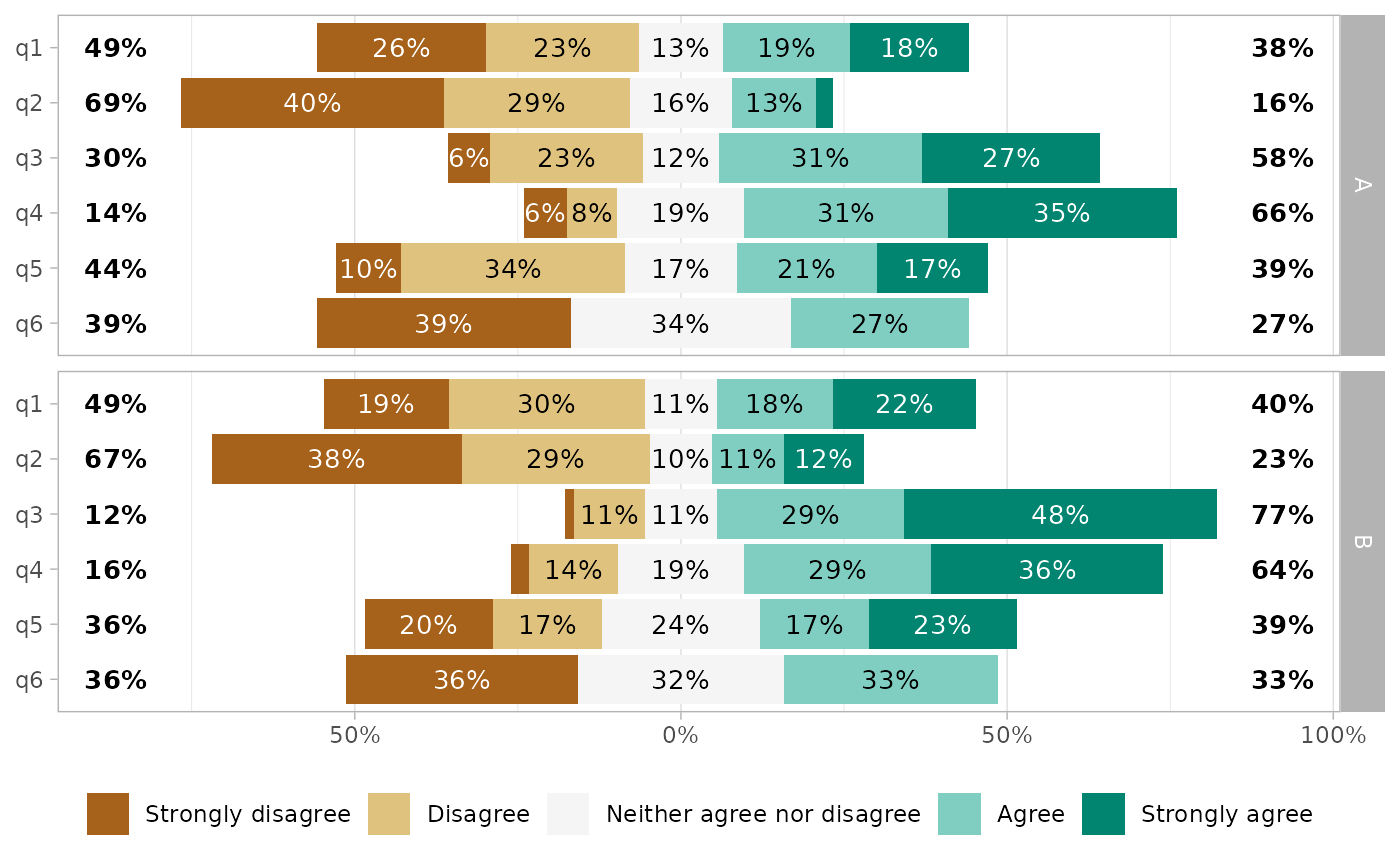gglikert(df_group, q1:q6, facet_cols = vars(group))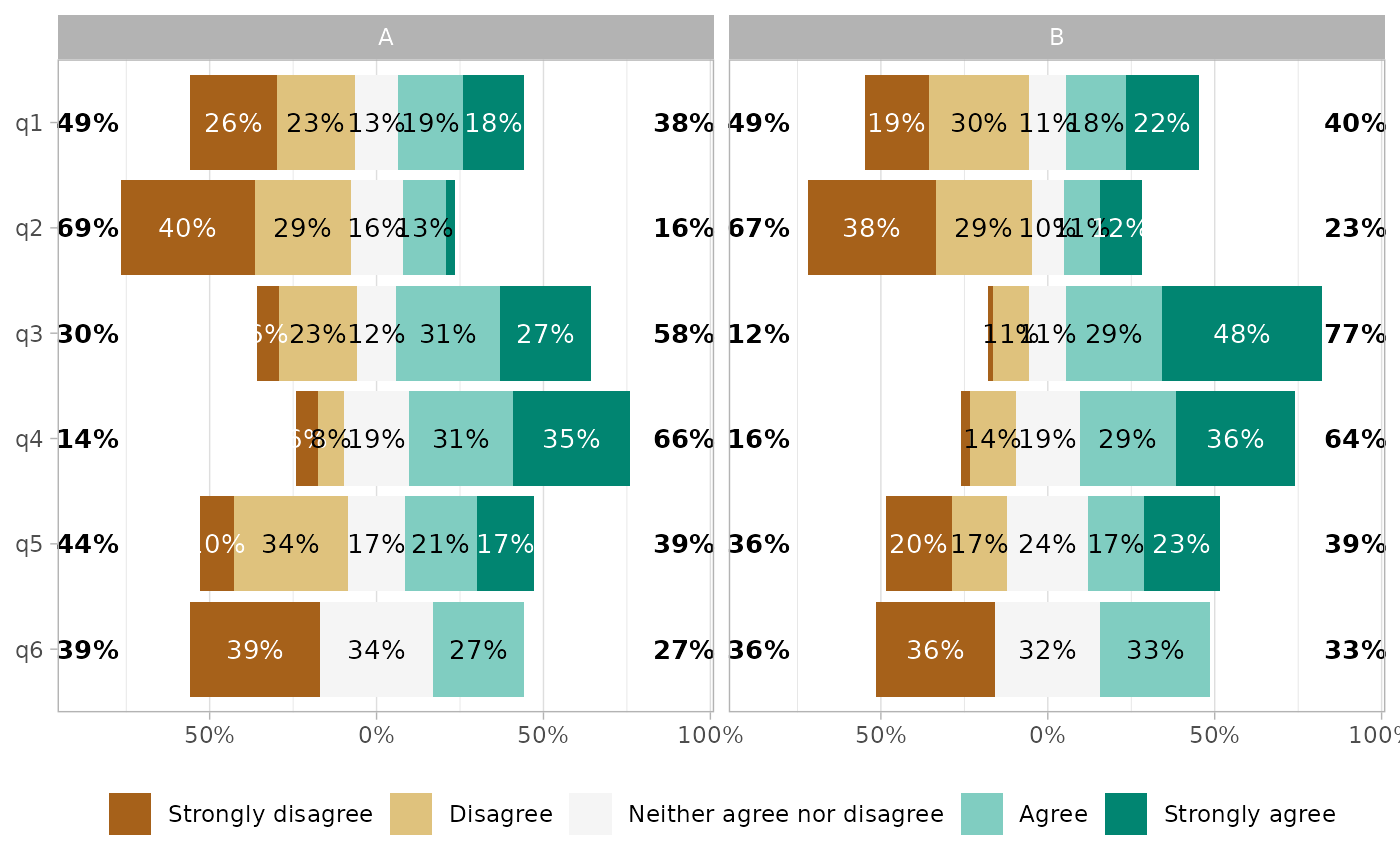gglikert(df_group, q1:q6, y = "group", facet_rows = vars(.question))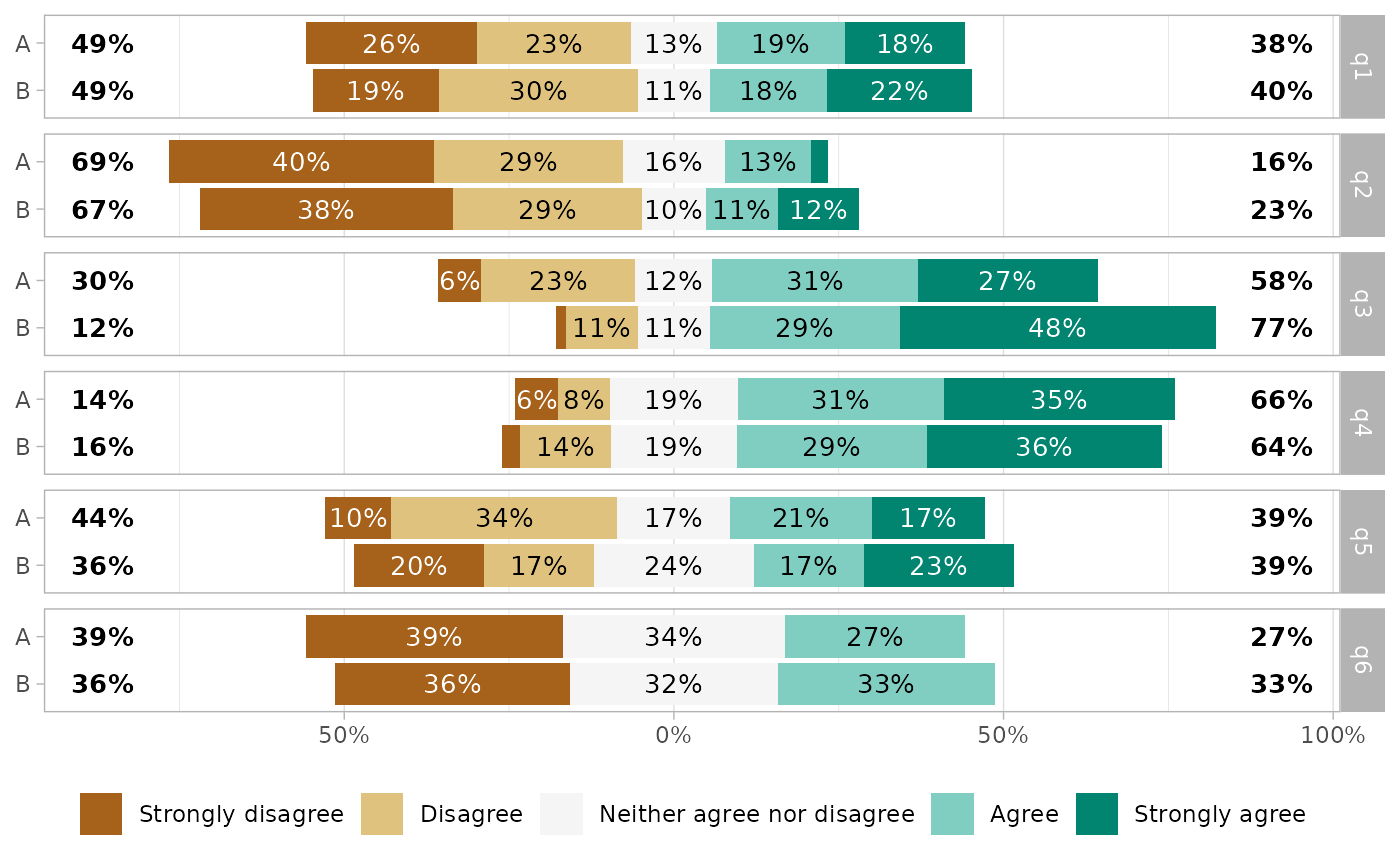# }
gglikert_stacked(df, q1:q6)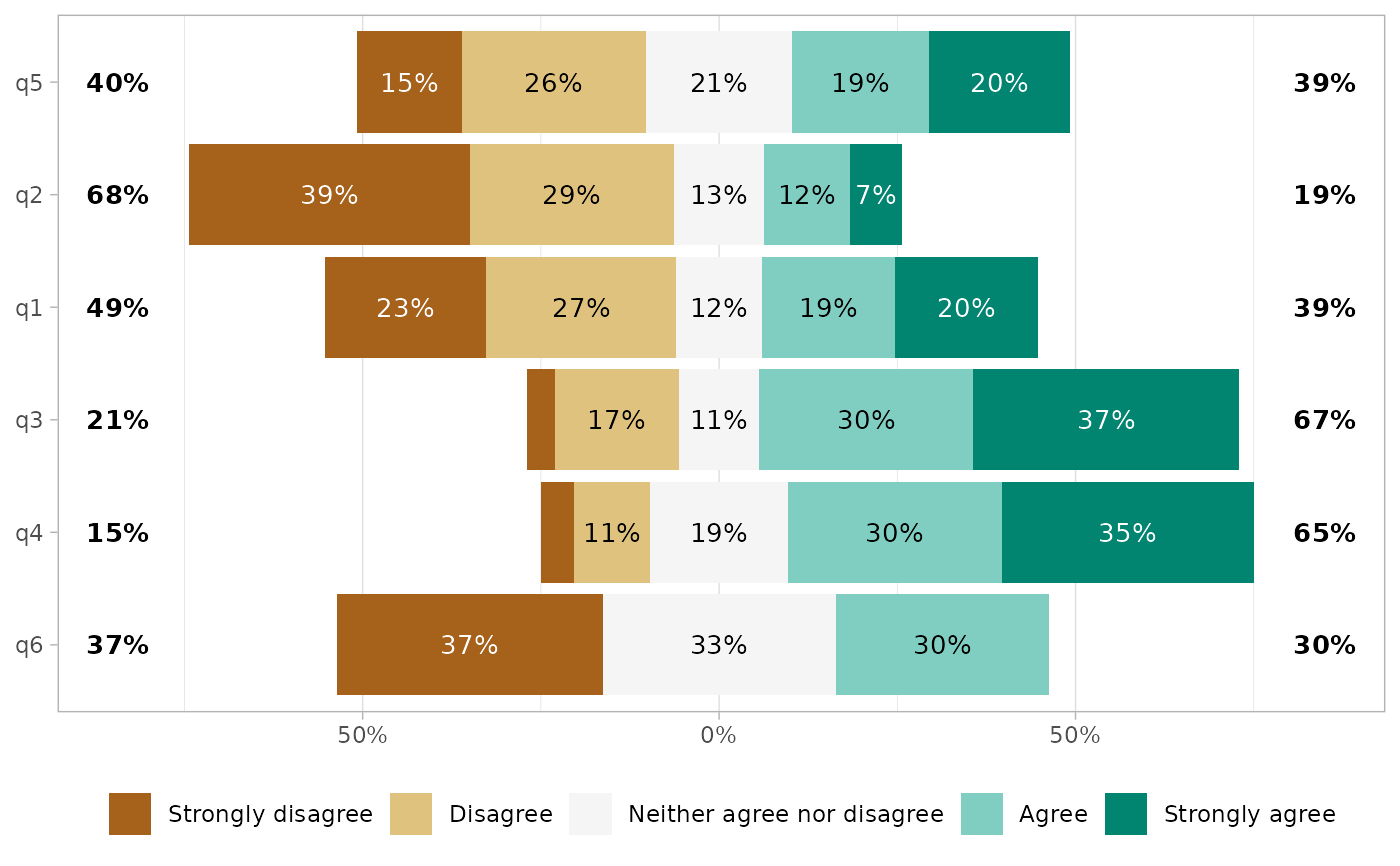gglikert_stacked(df, q1:q6, add_median_line = TRUE, sort = "asc")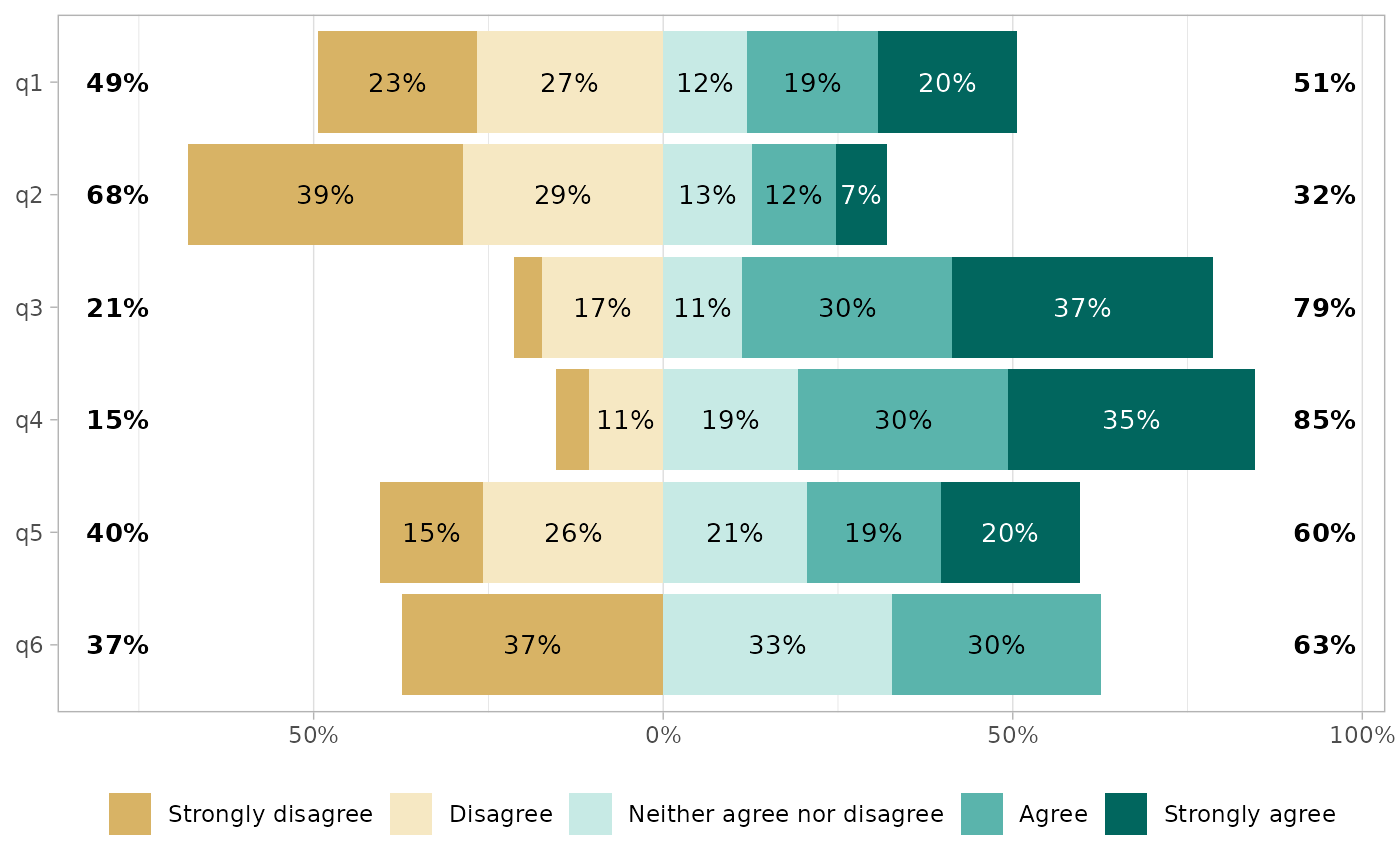# \donttest{
gglikert_stacked(df_group, q1:q6, y = "group", add_median_line = TRUE) +
facet_grid(rows = vars(.question))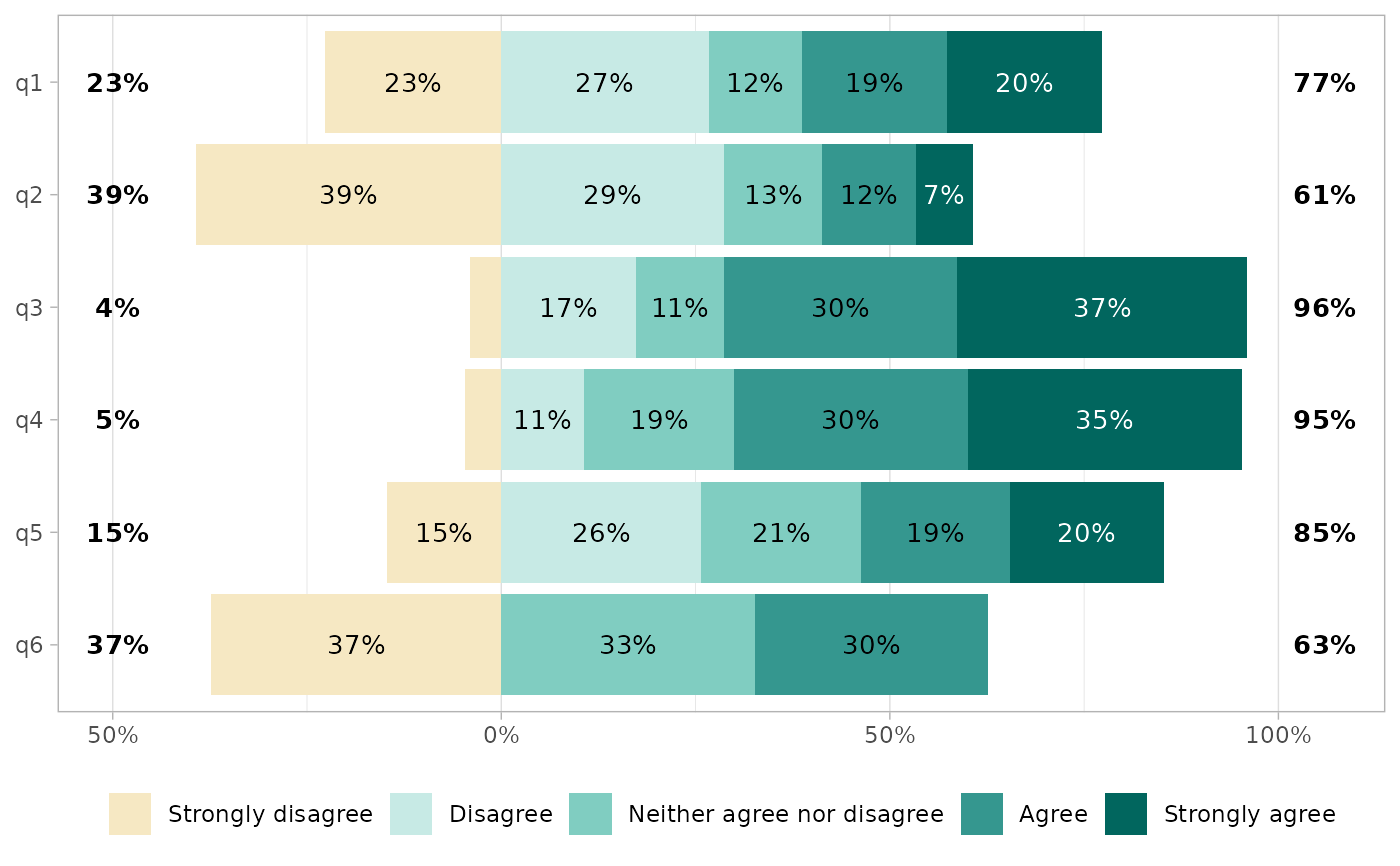# }